# Go Math Grade 3 Answer Key Chapter 5 Use Multiplication Facts Assessment Test

Go Math Grade 3 Answer Key Chapter 5 contains all the topics of chapter 5 which helps to test the student’s knowledge. Through this assessment test, students can check their knowledge. This assessment test is also helpful for the teachers to know how much a student understood the topics.

This chapter can improve student’s math skills, by referring to the Go Math Grade 3 Answer Key Chapter 5 Use Multiplication Facts Assessment Test, and with the help of this Go Math Grade 3 Assessment Test Answer Key, students can score good marks in the examination.

Chapter 5: Use Multiplication Facts Assessment Test

### Test – Page 1 – Page No. 51

Question 1.
The dance company wants to rent trunks to hold their costumes when they travel. Each trunk can hold costumes for 6 dancers. Which equation could be used to find how many trunks are needed for 48 dancers?
Options:
a. 6 × 48 = ■
b. 48 × ■ = 6
c. 48 × 6 = ■
d. ■ × 6 = 48

Answer: option d, 8 × 6 = 48

Explanation:
Given that trunk can hold costumes for 6 dancers
No of trunks needed to hold costumes of 48 dancers = 48 / 6 = 8
Therefore  8 × 6 = 48.

Question 2.
Select the equations that show the Distributive Property. Mark all that apply.
Options:
a. 3 × 60 = 3 × (20 + 20 + 20)
b. 5 × 20 = (5 × 2) × (5 × 10)
c. 50 × 7 = 7 × 50
d. 8 × (10 + 30) = 8 × 40

Explanation:
Both option a and d shows the Distributive Property,
3 × 60 = 3 × (20 + 20 + 20)
90 = 3 × (60) => 90, LHS = RHS. Same applies for option d as well.

Question 3.
Choose the number from the box that makes the sentence true.
Study hall has 63 desks. There are 9 desks in each row.
There arerows of desks in study hall.
_____

Answer: 7 × 9 = 63

Explanation:
Given that a study hall has 63 desks and each row consist of 9 desks
Therefore no of rows in study hall = 63/9 = 7.

### Test – Page 2 – Page No. 52

Question 4.
For numbers 4a-4d, choose True or False for each equation.
a. 9 × (3 + 3) = 9 × 6
i. True
ii. False

Explanation: 9 × (3 + 3) = 9 × 6 => 9 × 6 = 54, both L.H.S and R.H.S gives 54.

Question 4.
b. 9 × (4 + 4) = 9 × 6
i. True
ii. False

Explanation: 9 × (4 + 4) = 9 × 6 => 9 × 8 = 9 × 6 => 72 ≠ 54.

Question 4.
c. (3 × 9) + (2 × 9) = 9 × 6
i. True
ii. False

Explanation: (3 × 9) + (2 × 9) = 9 × 6 => 27 + 18 = 9 × 6 => 45 ≠ 54.

Question 4.
d. (9 × 4) + (9 × 2) = 9 × 6
i. True
ii. False

Explanation: (9 × 4) + (9 × 2) = 9 × 6 => 36 + 18 = 54 => 54 = 54, both L.H.S = R.H.S.

Question 5.
Carmen keeps her card collection in a folder with 20 pages. Each page has 8 cards. Willie has 150 cards in his collection. Does Carmen have more, fewer, or the same number of cards as Willie?
Options:
a. She has more cards than Willie.
b. She has the same number of cards as Willie.
c. She has fewer cards than Willie.

Explanation: Carmen has 20 pages folder each having 8 cards, therefore total cards = 20 × 8 = 160
Given that Willie has 150 cards, Therefore Carmen has 10 more cards compared to Willie.

Question 6.
For items 6a–6d, choose Yes or No to show whether the unknown factor is 9.
a. 6 × ■ = 56
i. yes
ii. no

Explanation: 6 × 9 = 54 not 56.

Question 6.
b. ■ × 4 = 42
i. yes
ii. no

Explanation: 9 × 4 = 36 not 42.

Question 6.
c. 8 × ■ = 72
i. yes
ii. no

Explanation: 8 × 9 = 72.

Question 6.
d. ■ × 50 = 450
i. yes
ii. no

Explanation: 9 × 50 = 450.

Question 7.
Each bus can carry 40 people. Use the number line to find how many people 3 buses can carry._____ people

Explanation: From below number line 3 buses carry = (40 + 40 +40) = 120 people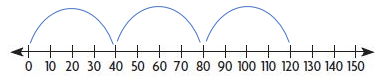### Test – Page 3 – Page No. 53

Question 8.
Neil made this multiplication model. Complete the equation that represents the model.
_____ × _____ = _____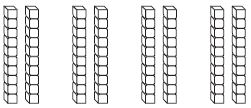Answer: 20 × 4 = 80

Explanation:
In the given model every column contains 10 boxes in each row.
Every part has 2 rows, so each part contains 20 boxes
Since we have 4 parts of it total boxes would be = 20 × 4 = 80
Therefore equation that represents the multiplication model is 20 × 4 = 80.

Question 9.
A flower shop delivers fresh flower bouquets to hotels each week. Which hotel buys the least number of flowers?__________

Explanation:
No of flowers in 1 Bouquet of Welcome Inn Hotel = 7
No of Bouquets = 50, total no of flowers = 7 × 50 = 350
No of flowers in 1 Bouquet of Wild Rose Hotel = 8
No of Bouquets = 60, total no of flowers = 8 × 60 = 480
No of flowers in 1 Bouquet of Bayside Hotel = 6
No of Bouquets = 70, total no of flowers = 6 × 70 = 420
No of flowers in 1 Bouquet of Bayside Hotel = 5
No of Bouquets = 80, total no of flowers = 6 × 80 = 480
Therefore comparing all the hotels, Welcome Inn Hotel buys the least number of flowers.

Question 10.
A store has 3 crates of oranges. Each crate holds 2 boxes. Each box holds 20 oranges. What is the total number of oranges in the store?
_____ oranges

Answer: Total no of oranges in the store is 120.

Explanation:
Given that store has 3 crates of oranges having 2 boxes each
Each box holds 20 oranges, total no of oranges = 3 × 2 × 20 = 120.

Question 11.
The new lion cub at the zoo weighs 41 pounds. He has been gaining 5 pounds every month as he grows. If this pattern continues, how much will the cub weigh 4 months from now?
_____ pounds

Explanation:
Weight of lion cub = 41 pounds
Number of pounds increases every month = 5
Current weight of cub from past 4 months = 41 + (4 × 5) = 61.

### Test – Page 4 – Page No. 54

Question 12.
Shelly describes a pattern. She says the pattern shown in the table is “Add 5.” Is Shelly correct? Explain how you know.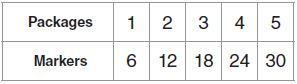_____

Answer: No, she is not correct

Explanation: The pattern works for the first pair of (packages and markers) numbers, not for the remaining. The pattern is “Multiply the number of packages by 6.”

Question 13.
This shows a part of a multiplication table. Find the missing numbers. Explain how you found the numbers.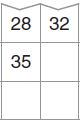Explanation:
The only numbers that have a product of 28 are 4 and 7.
The only numbers that have a product of 32 are 4 and 8.
This tells us that 4 is the number for the top row. That means 5 is the next row down and 6 is the row after that.
The factors of the columns are 7 and 8, so we can multiply to find the missing numbers.

Question 14.
Describe a pattern for this table.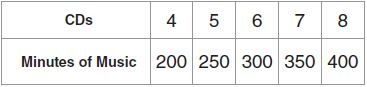Pattern: _________________

Answer: Multiply the number of CDs by 50.

How would the table change if the pattern was “Multiply the number of CDs by 5”? Explain.

Answer: The table would change by taking a zero off each number of minutes in the second row because you would be multiplying by ones, not tens.

### Test – Page 5 – Page No. 55

Question 15.
Aroon has 180 cat toys to pack in boxes. He packs 30 toys in each box. How many boxes does he need?
Write an equation using the letter n to stand for the unknown factor. Explain how to find the unknown factor.

Explanation:
Aroon has 180 cat toys, he packs 30 toys in each box
Let no of boxes needed be n
therefore n × 30 = 180 => n = 180/30 = 6.

Question 16.
A store has 5 shelves of soup. There are 20 cans of soup on each shelf. How many cans of soup does the store have?
Shade squares to make a diagram to show how you can use the Distributive Property to find the number of cans of soup in the store._____ cans of soup

Answer: 5 × (10 + 10) = (5 × 10) + (5 × 10) = 50 + 50 = 100

Explanation:
Given that store has 5 shelves of Soup, each shelf has 20 cans of soup.
Therefore the number of can of soup store have = 5 × 20 = 100 cans
From the given model we can write 5 × (10 + 10) = (5 × 10) + (5 × 10) = 100.### Test – Page 6 – Page No. 56

Question 17.
Beth saves all her dimes. Today she is getting them out of her coin jar and wrapping them to take to the bank. She finds she has 400 dimes. It takes 50 dimes to fill each paper wrapper and make a roll. How many wrappers does she need?
Part A
Write an equation using n for the unknown factor. Find the number of wrappers needed.
_____ × _____ = _____
_____ × _____ = _____

n × 50 = 400
n = 400/50 = 20 wrappers

Explanation:
Given that Beth has 400 dimes and in each paper wrapper she fills 50 dimes
No of paper wrappers needed = n
therefore n = 400/50 = 20.

Question 17.
Part B
Explain how you solved this problem and how you know your answer is correct.

Explanation:
We used place value, and we know 8×5= 40, so 8×5 tens= 40 tens. As 40 tens = 400.

Question 18.
Two friends have jobs at the mall. Juan works 40 hours each week and earns $9 per hour. Thomas works 50 hours per week and earns$7 per hour.
Part A
Who earns more each week?

Answer: Juan earns for each week compared to Thomas.

Explanation:
Juan works for 40 hrs each week earning $9 per hr Total earnings of Juan per week = 40 × 9 =$ 360
Thomas works for 50 hrs each week earning $7 per hr Total earnings of Thomas per week = 50 × 7 =$ 350.

Question 18.
Part B
Juan works for 40 hrs each week earning $9 per hr Total earnings of Juan per week = 40 × 9 =$ 360
Thomas works for 50 hrs each week earning $7 per hr Total earnings of Thomas per week = 50 × 7 =$ 350.